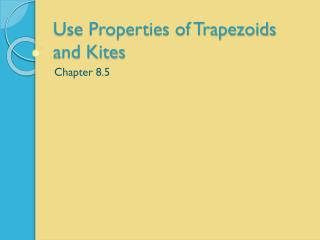# Use Properties of Trapezoids and Kites - PowerPoint PPT PresentationDownload PresentationUse Properties of Trapezoids and Kites

Use Properties of Trapezoids and KitesDownload Presentation## Use Properties of Trapezoids and Kites

- - - - - - - - - - - - - - - - - - - - - - - - - - - E N D - - - - - - - - - - - - - - - - - - - - - - - - - - -
##### Presentation Transcript

1. Use Properties of Trapezoids and Kites Chapter 8.5

2. Trapezoids • Trapezoids are quadrilaterals that have 2 parallel sides • The parallel sides are called the bases. • A trapezoid has 1 pair of base angles. • The non-parallel sides are called the legs. base Leg Leg base

3. Is it a trapezoid? Are the bases parallel? Find the slope of each base. If the slopes are the same, then it is a trapezoid.

4. Isosceles Trapezoids • Isosceles triangles have 2 congruent sides with 2 pairs of congruent angles. • The diagonals of an isosceles trapezoid are also congruent.

5. If it is Isosceles find the missing angles. It is isosceles! 53º 127º 127º Because it is an isosceles trapezoid, the base angles are congruent. Therefore m <A = m <B, and m <D = m <C Angle A and Angle D are supplementary. 180 – 53 = 127

6. Midsegments • A midsegment is a segment that connects 2 midpoints. • The midsegment of a trapezoid connects the midpoints of the legs.

7. Find the length of the midsegment

8. Things always have to be more difficult 19.5 5x + 3

9. Find x 45 52.5 10x + 15

10. Page 546, #3 – 15, 25 - 27

11. Kites • A kite is a quadrilateral with 2 pairs of congruent sides, but the opposite sides are not congruent.

12. Diagonals If the diagonals of a kite are perpendicular, then what shape is created by the diagonals? If we are given these side lengths, can we find the missing sides XY, WX, YZ , and WZ?

13. Find XY, ZY, WX, and WZ 2√13 6√5

14. Find XY, YZ, WZ, and WX 5√5 √461

15. The figure below is a kite, find the missing angles What is the sum of the interior angles of a kite? 360 100 + 40 + mó E + mó G = 360 140 + mó E + mó G = 360 mó E + mó G = 220 What do we know about the measures of the angles E and G? They are congruent!

16. Find the missing angles What do we know about the measures of the angles F and H? They are congruent! 110º 60 + 110 + 110 + mó G = 360 80º 280 + mó G = 360 mó G = 80

17. Find the missing angles 150 + 90 + mó F + mó G = 360 240 + mó F + mó G = 360 mó F + mó G = 120 mó F = mó G